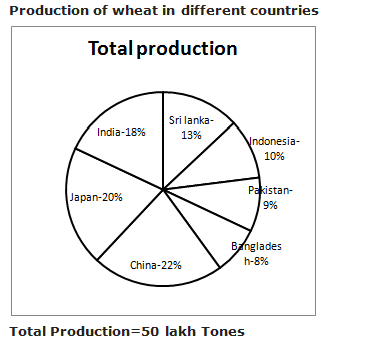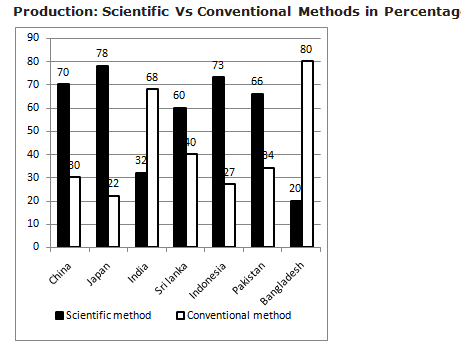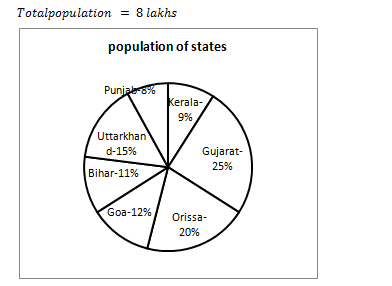# Important Data Interpretation Set – 99

D.1-5) Study the following graph carefully to answer the questions:

Production of wheat in different countriesTotal Production=50 lakh Tonnes

Production: Scientific Vs Conventional Methods in PercentageQ.1) What is the difference between the production by scientific method and conventional method in Indonesia?

a)  2.3 lakh tonnes

b) 2.8 lakh tones

c) 2.5 lakh tonnes

d) 3 lakh tones

e) None of these

a)  2.3 lakh tonnes

Production In Indonesia= 50 x 10/100= 5 lakh tones

Difference= 5 x ((73 – 27))/100= 2.3 lakh tones

Q.2) What is the average production of wheat by scientific method for all the countries?

a) 3.1 lakh tonnes

b) 4.24 lakh tones

c) 2.6 lakh tonnes

d) 3.07 lakh tones

e) None of these

b) 4.24 lakh tones

Production of wheat by scientific method in

India= 50 x (18/100)x (32/100)= 2.88 lakh tones

Japan = 50 x (20/100)x (78/100)= 7.8 lakh tones

China= 50 x (22/100)x (70/100)= 7.7 lakh tones

Bangladesh= 50 x (8/100)x (20/100)= 0.8 lakh tones

Pakistan= 50 x (9/100)x (66/100)= 2.97 lakh tones

Indonesia= 50 x (10/100)x (73/100)= 3,65 lakh tones

Sri Lanka= 50 x (13/100)x (60/100)= 3.9 lakh tones

So Average = (2.88 + 7.8 + 7.7 + 0.8 + 2.97 + 3.65 + 3.9)/7= 4.24 lakh tones

Q.3) What is the ratio of production by conventional method in Pakistan to that by scientific method in Japan?

a)  9/40

b) 99/260

c) 51/260

d) 48/77

e) None of these

c) 51/260

Production by conventional method in Pakistan = 50 x (9/100)x (34/100)= 1.53 lakh tones

Production by scientific method in Japan= 7.8 lakh tones

Required ratio= 1.53 : 7.8 = 51 : 260

Q.4) The production of wheat in Sri Lanka by Conventional method is approximately how many times the production in India by scientific method?

a) 1.2

b) 1.5

c) 0.9

d) 2.1

e) None of these

c) 0.9

Production of wheat in Sri Lanka by Conventional method= 50 x (13/100)x (40/100)= 2.6 lakh tones

Production in India by scientific method= 50 x (18/100)x (32/100)= 2.88 lakh tones

According to question, 2.88 X x = 2.6

x = 2.6/2.88= 0.9 times

Q.5) The production of wheat in India by Scientific method is approximately how many times the production in Pakistan by Conventional method?

a)  2.88

b) 2.07

c) 2.61

d) 0.81

e) None of these

e) None of these

Production of wheat in India by Scientific method= 50 x (18/100)x (32/100)= 2.88 lakh tones

Production of wheat in Pakistan by Conventional method=50×9/100×34/100=1.53

=2.88/1.53=1.88

D.6-10) Study the following graph carefully to answer the questions:

Data of different states regarding population of states in the year 1991Table shows the ratio of male to female graduates in different states and percentage of people non graduates

 States Ratio of Male to female graduates Percentage of non-graduates Male Female Kerala 3 2 20% Gujarat 1 3 35% Orissa 3 5 25% Goa 2 3 15% Bihar 3 1 20% Uttarkhand 5 1 30% Punjab 7 5 25%

Q.6) In which state has highest number of female graduates in all the states together?

a) Punjab

b) Gujarat

c) Goa

d) Kerala

e) Bihar

b) Gujarat

Kerala=9/100×8 lakh=72000

Gujarat=25/100×8 lakh=200000

Orissa=20/100×8lakh=160000

Goa=12/100×8lakh=96000

Bihar=11/100×8lakh=88000

Uttarkhand=15/100×8lakh=120000

Punjab=8/100×8lakh=64000

Kerala=80/100×72000=57600

Gujarat=65/100×200000=130000

Orissa=75/100×160000=120000

Goa=85/100×96000=81600

Bihar=80/100×88000=70400

Uttarkhand=70/100×120000=84000

Punjab=75/100×64000=48000

Number of female graduates in all the states

Kerala=57600×2/5=23040

Gujarat=130000×3/4=97500

Orissa=120000×5/8=75000

Goa=81600×3/5=48960

Bihar=70400×1/4=17600

Uttarkhand=84000×1/6=14000

Punjab=48000×5/12=20000

In the state Gujarat has maximum female graduates.

Q.7) What is the difference between the number of female graduates in Bihar, Orissa and Punjab together and the number of male graduates in the state Uttarkhand, Goa and Gujarat together?

a) 28000

b) 27605

c) 26080

d) 22540

e) None of these

d) 22540

Bihar=80/100×88000=70400

Punjab=75/100×64000=48000

Orissa=75/100×160000=120000

Gujarat=65/100×200000=130000

Goa=85/100×96000=81600

Uttarkhand=70/100×120000=84000

Orissa=120000×5/8=75000

Bihar=70400×1/4=17600

Punjab=48000×5/12=20000

Uttarkhand=84000×5/6=70000

Goa=81600×2/5=32640

Gujarat=130000×1/4=32500

Required difference=(75000+17600+20000)-(70000+32640+32500)

=135140-112600=22540

Q.8) If the total population of all the states 20% increased in the year 1992 compared to the previous year and all other data are same in the year 1991, approximately What percentage of graduate male in Bihar is the graduates female in Orissa?

a) 75%

b) 80%

c) 70%

d) 72%

e) None of these

c) 70%

Total population in the year 1992= 120/100×8lakh=9.6 lakh

Population in Bihar=11/100×9.6=105600

Population in Orissa=20/100×9.6=192000

Reqired percentage=63360/90000×100=70%

Q.9) If the ratio of non-graduates male to female is 1:3 in Bihar, Punjab, Kerala and Gujarat, the number of male non graduates in Bihar and Punjab together is approximately what percentage more/less the number of non-female graduates in Kerala and Gujarat together?

a) 87%

b) 96%

c) 88%

d) 84%

e) None of these

a) 87%

Total population in given states,

Kerala=9/100×8 lakh=72000

Gujarat=25/100×8 lakh=200000

Bihar=11/100×8lakh=88000

Punjab=8/100×8lakh=64000

Bihar=88000×20/100×1/4=4400

Punjab=64000×25/100×1/4=4000

Kerala=72000×20/100×3/4=10800

Gujarat=200000×35/100×3/4=52500

Required percentage=((52500+10800)-(4400+4000))/63300×100=86.7% ~ 87%

Q.10) What is the difference between the number of male graduates in all the states together and the number of female graduates in all the states together?

a) 650

b) 600

c) 700

d) 680

e) None of these

Kerala=9/100×8 lakh=72000

Gujarat=25/100×8 lakh=200000

Orissa=20/100×8lakh=160000

Goa=12/100×8lakh=96000

Bihar=11/100×8lakh=88000

Uttarkhand=15/100×8lakh=120000

Punjab=8/100×8lakh=64000

Kerala=80/100×72000=57600

Gujarat=65/100×200000=130000

Orissa=75/100×160000=120000

Goa=85/100×96000=81600

Bihar=80/100×88000=70400

Uttarkhand=70/100×120000=84000

Punjab=75/100×64000=48000

Number of female graduates in all the states

Kerala=57600×2/5=23040

Gujarat=130000×3/4=97500

Orissa=120000×5/8=75000

Goa=81600×3/5=48960

Bihar=70400×1/4=17600

Uttarkhand=84000×1/6=14000

Punjab=48000×5/12=20000

Kerala=57600×3/5=34560

Gujarat=130000×1/4=32500

Orissa=120000×3/8=45000

Goa=81600×2/5=32640

Bihar=70400×3/4=52800

Uttarkhand=84000×5/6=70000

Punjab=48000×7/12=28000

Required difference

=(34560+32500+45000+32640+52800+70000+28000)-(20000+14000+17600+48960+23040+97500+75000)

=295500-296100=600